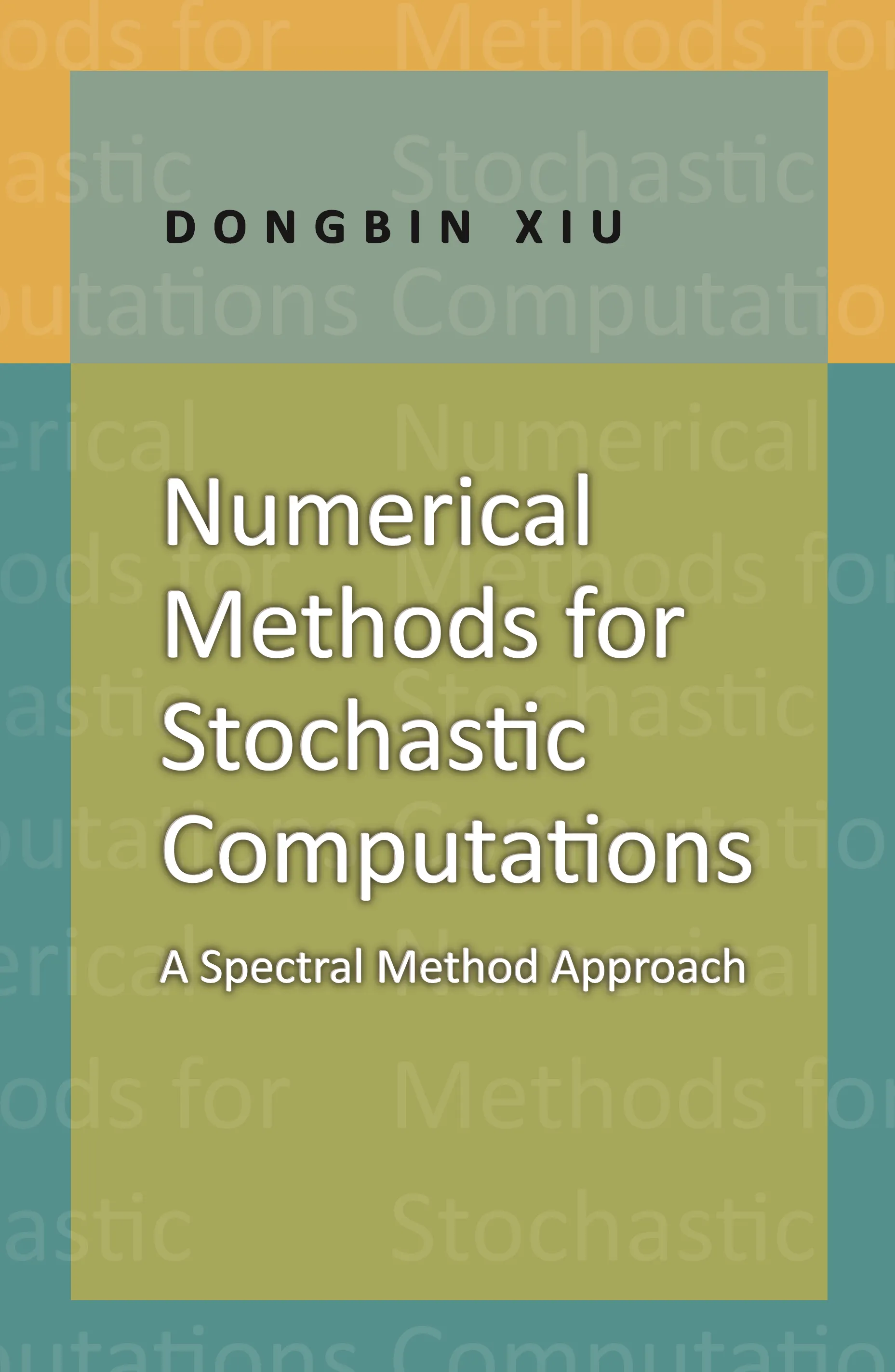#### Numerical Methods for Stochastic Computations

Dongbin Xiu

##### Before you purchase audiobooks and ebooks

Please note that audiobooks and ebooks purchased from this site must be accessed on the Princeton University Press app. After you make your purchase, you will receive an email with instructions on how to download the app.

# Numerical Methods for Stochastic Computations: A Spectral Method Approach## Hardcover

Price:
\$68.00/£58.00
ISBN:
Published:
Jul 21, 2010
2010
Pages:
144
Size:
6 x 9.25 in.
Illus:
50 line illus.

The@ first graduate-level textbook to focus on fundamental aspects of numerical methods for stochastic computations, this book describes the class of numerical methods based on generalized polynomial chaos (gPC). These fast, efficient, and accurate methods are an extension of the classical spectral methods of high-dimensional random spaces. Designed to simulate complex systems subject to random inputs, these methods are widely used in many areas of computer science and engineering.

The book introduces polynomial approximation theory and probability theory; describes the basic theory of gPC methods through numerical examples and rigorous development; details the procedure for converting stochastic equations into deterministic ones; using both the Galerkin and collocation approaches; and discusses the distinct differences and challenges arising from high-dimensional problems. The last section is devoted to the application of gPC methods to critical areas such as inverse problems and data assimilation.

Ideal for use by graduate students and researchers both in the classroom and for self-study, Numerical Methods for Stochastic Computations provides the required tools for in-depth research related to stochastic computations.

• The first graduate-level textbook to focus on the fundamentals of numerical methods for stochastic computations

• Ideal introduction for graduate courses or self-study

• Fast, efficient, and accurate numerical methods

• Polynomial approximation theory and probability theory included

• Basic gPC methods illustrated through examples